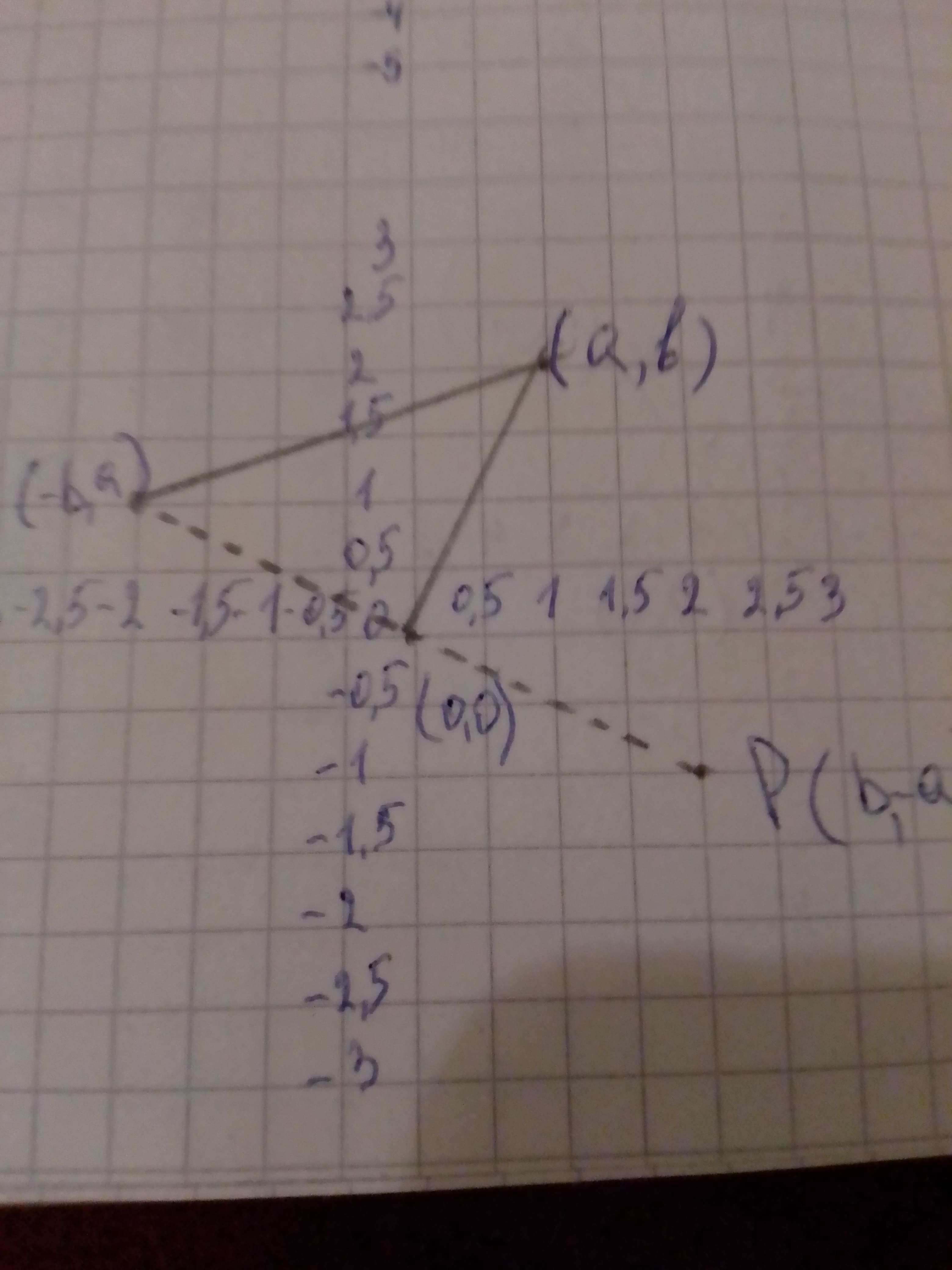# a) To find: The images of the following points under under a 90^circ rotation counterclockwise about the origin: I. (2, 3) II. (-1, 2) III, (m,n) inteTammy Todd 2020-12-01 Answered

a) To find:
The images of the following points under under a ${90}^{\circ }$ rotation counterclockwise about the origin:
I.
II.
III, (m,n) interms of m and n
b)To show:
That under a half-turn with the origin as center, the image of a point
c) To find:
The image of about the origin.

You can still ask an expert for help

• Questions are typically answered in as fast as 30 minutes

Solve your problem for the price of one coffee

• Math expert for every subject
• Pay only if we can solve itdessinemoie

a) Given:
The image will have a ${90}^{\circ }$ rotation counterclockwise a about the origin.
Approach:
A rotation is a transformation of the plane determined by holding one point the center fixed and rotating the plane about this point by a certain angle clockwise (negative) or counterclockwise (positive).
Calculation:
I.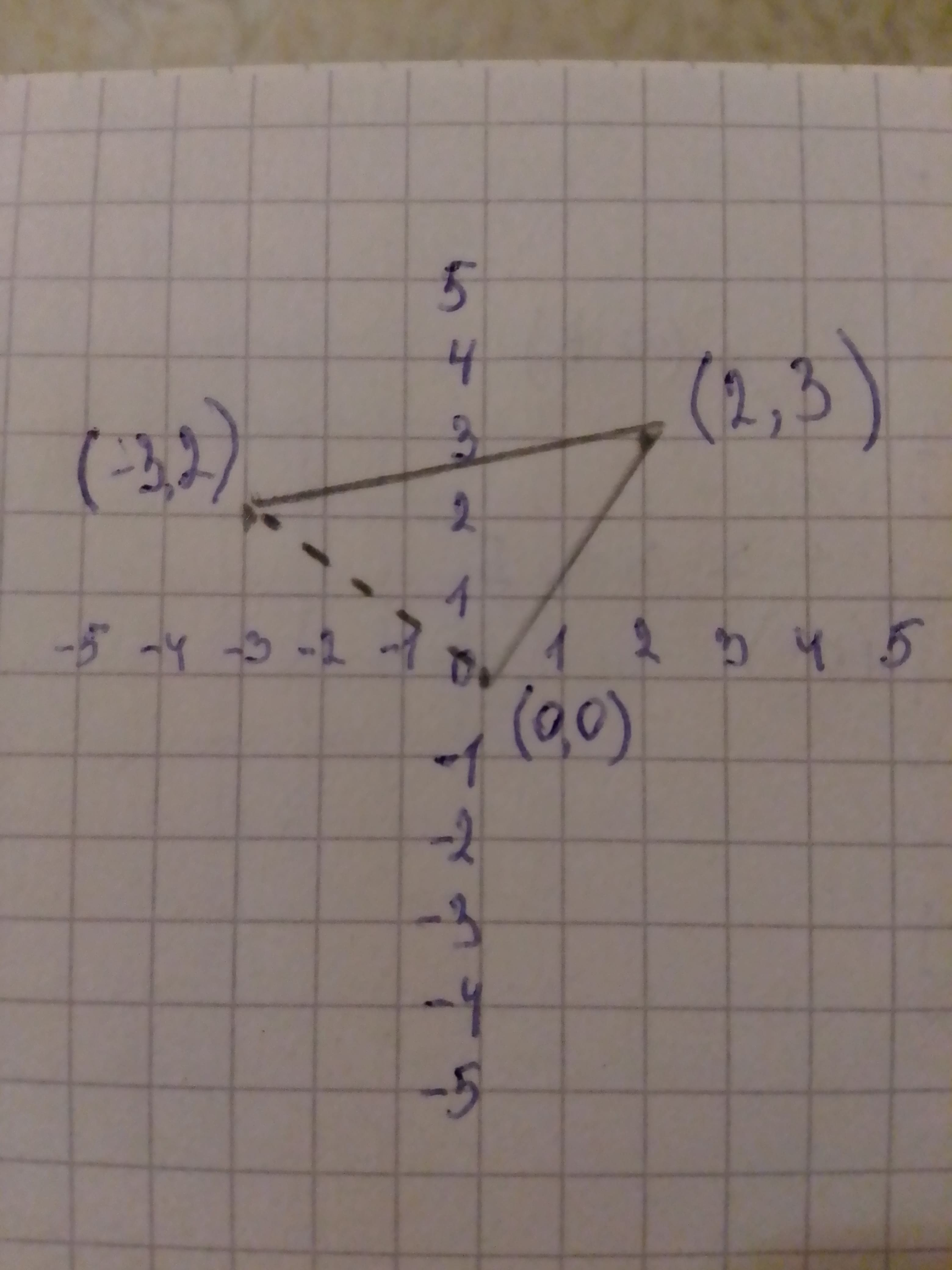II.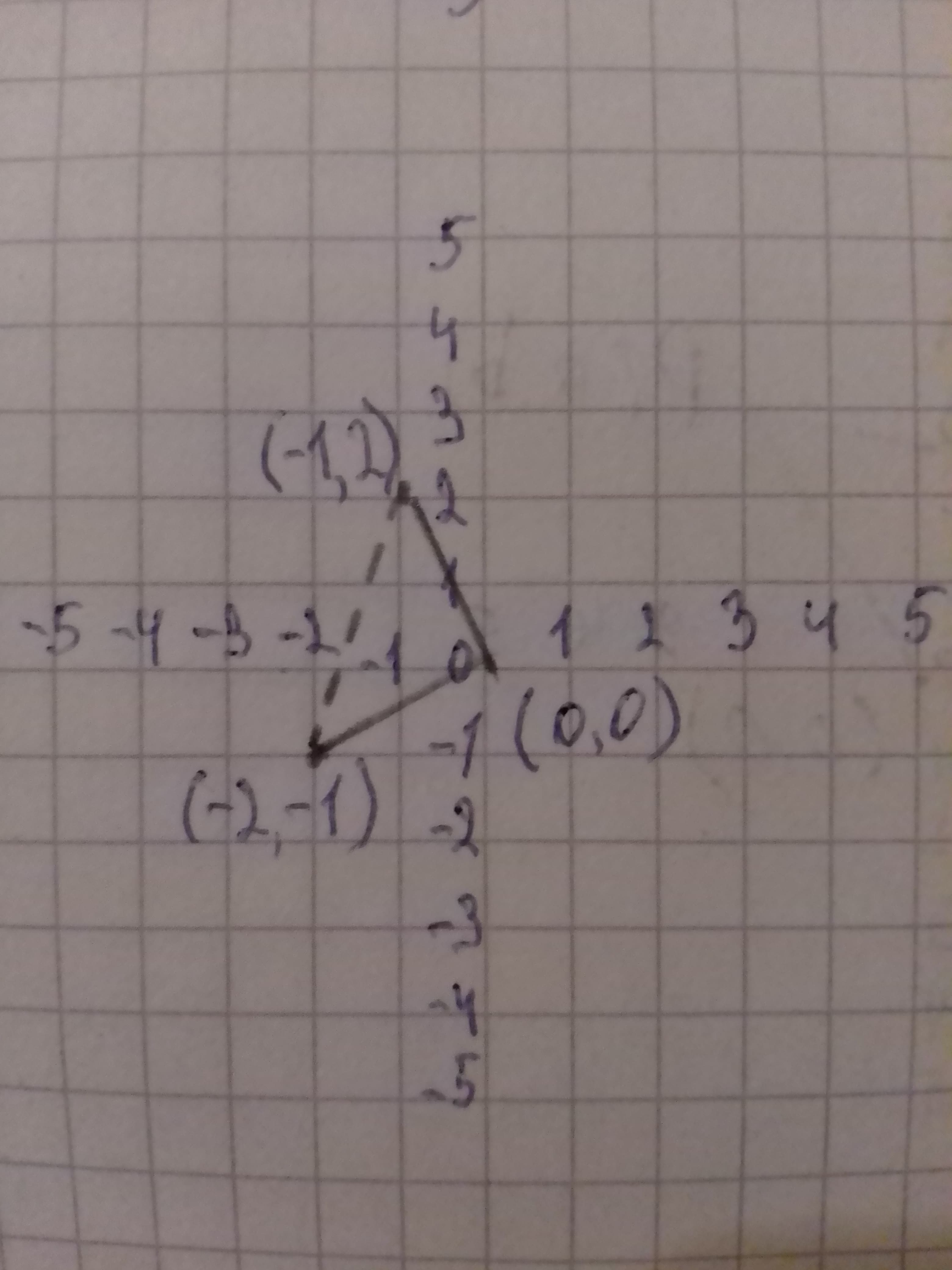III. interms of m and n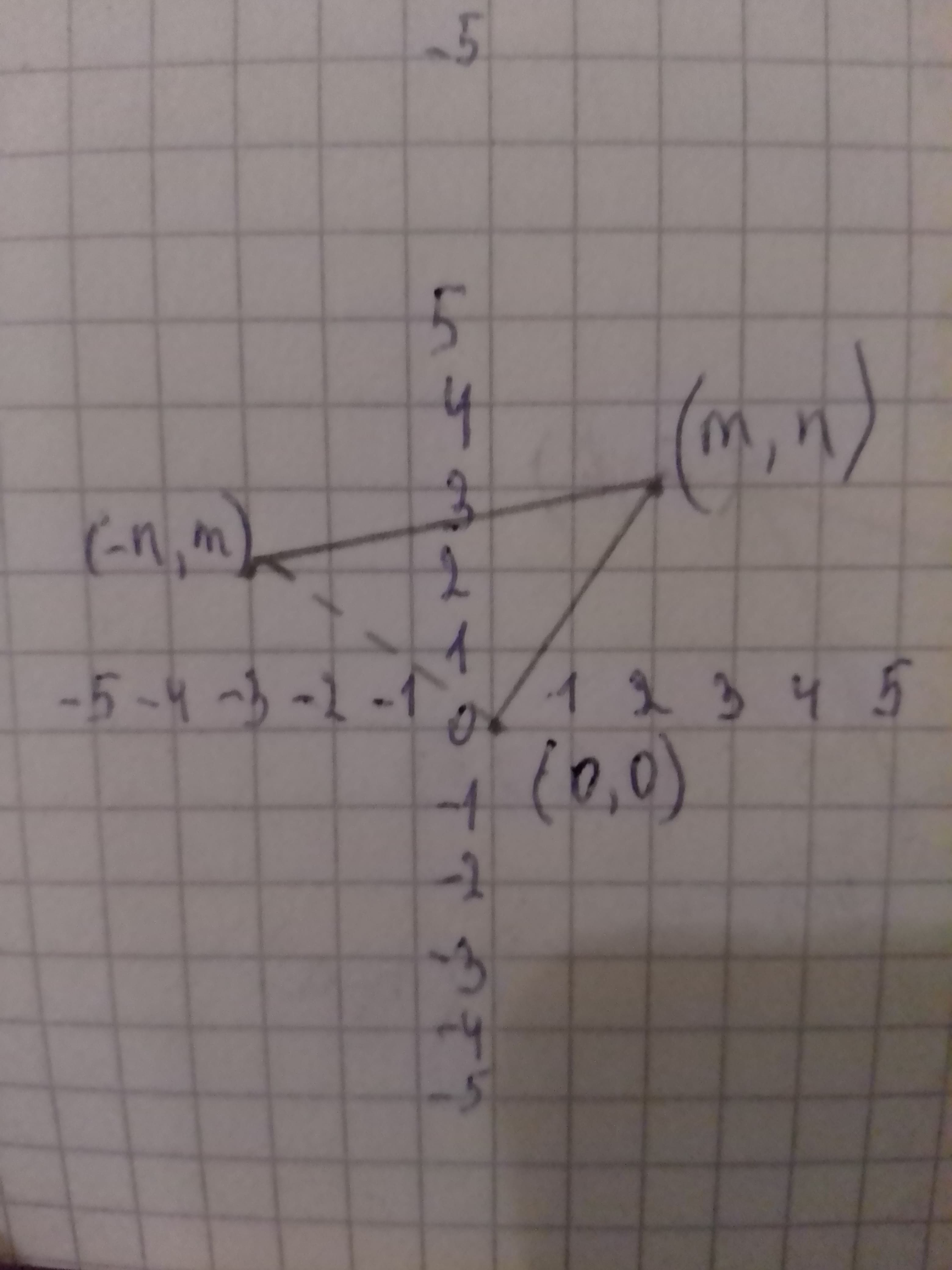b) Given:
Under a half-turn with the origin as center the image of a point
Approach:
A rotation is a transformation of the plane determined by holding one point-the center-fixed and rotating the plane about this point by a certain angle clockwise (negative) or counterclockwise (positive).
Calculation:
While taking half-turn rotation with center at the origin for as shown in the graph below.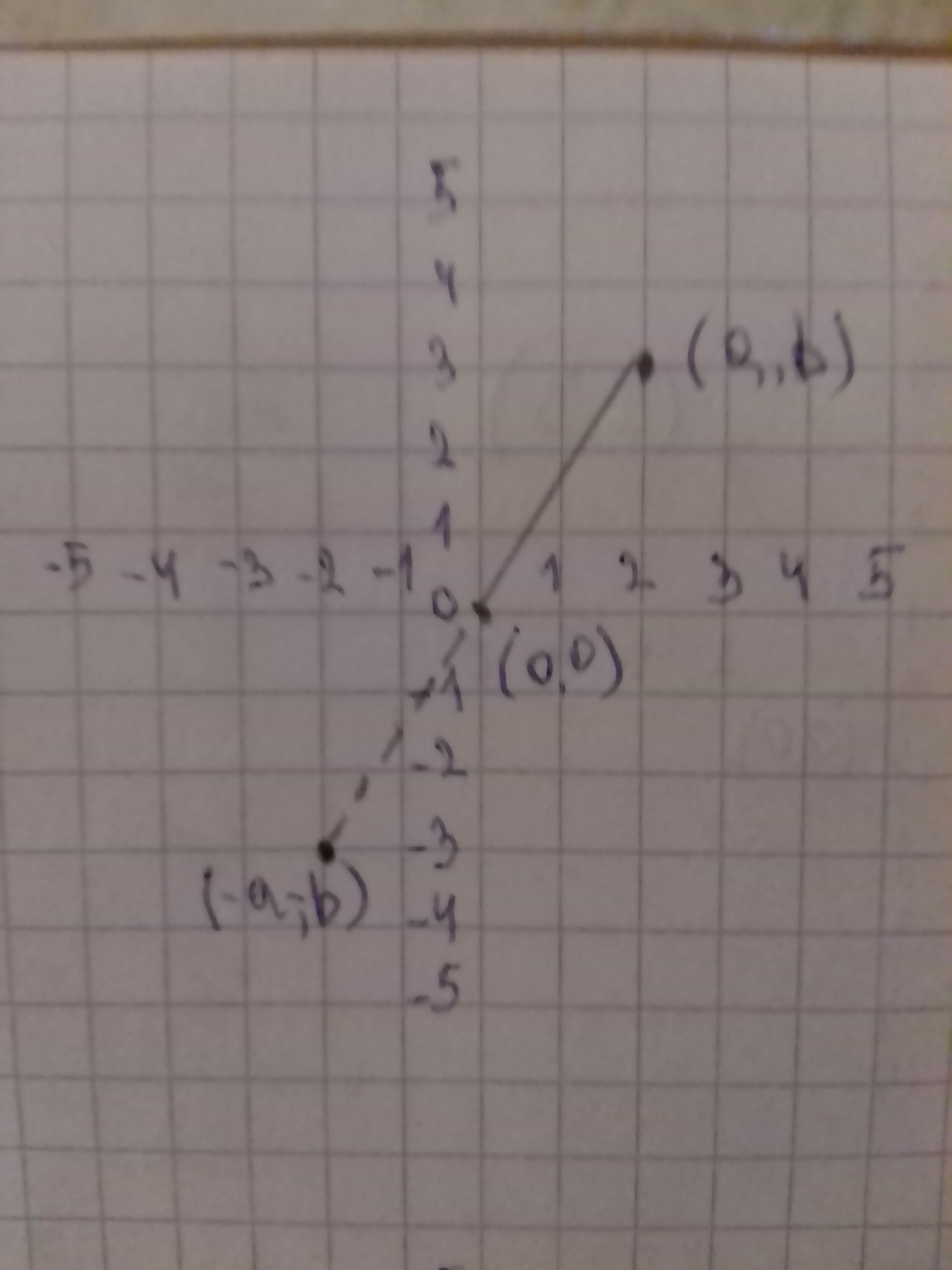c) Given:
A point
Approach:
A rotation is a transformation of the plane determined by holding one point-the center-fixed and rotating the plane about this point by a certain angle clockwise (negative) or counterclockwise (positive).
Calculation:
The rotation of ${180}^{\circ }$ about a point is a half-turn is a rotation thus it has all the properties of rotations.
The image of i.e., clockwise.
The graphical representation is as under:
As given in question to take the reference from point (a) for turn of ${90}^{\circ ,}$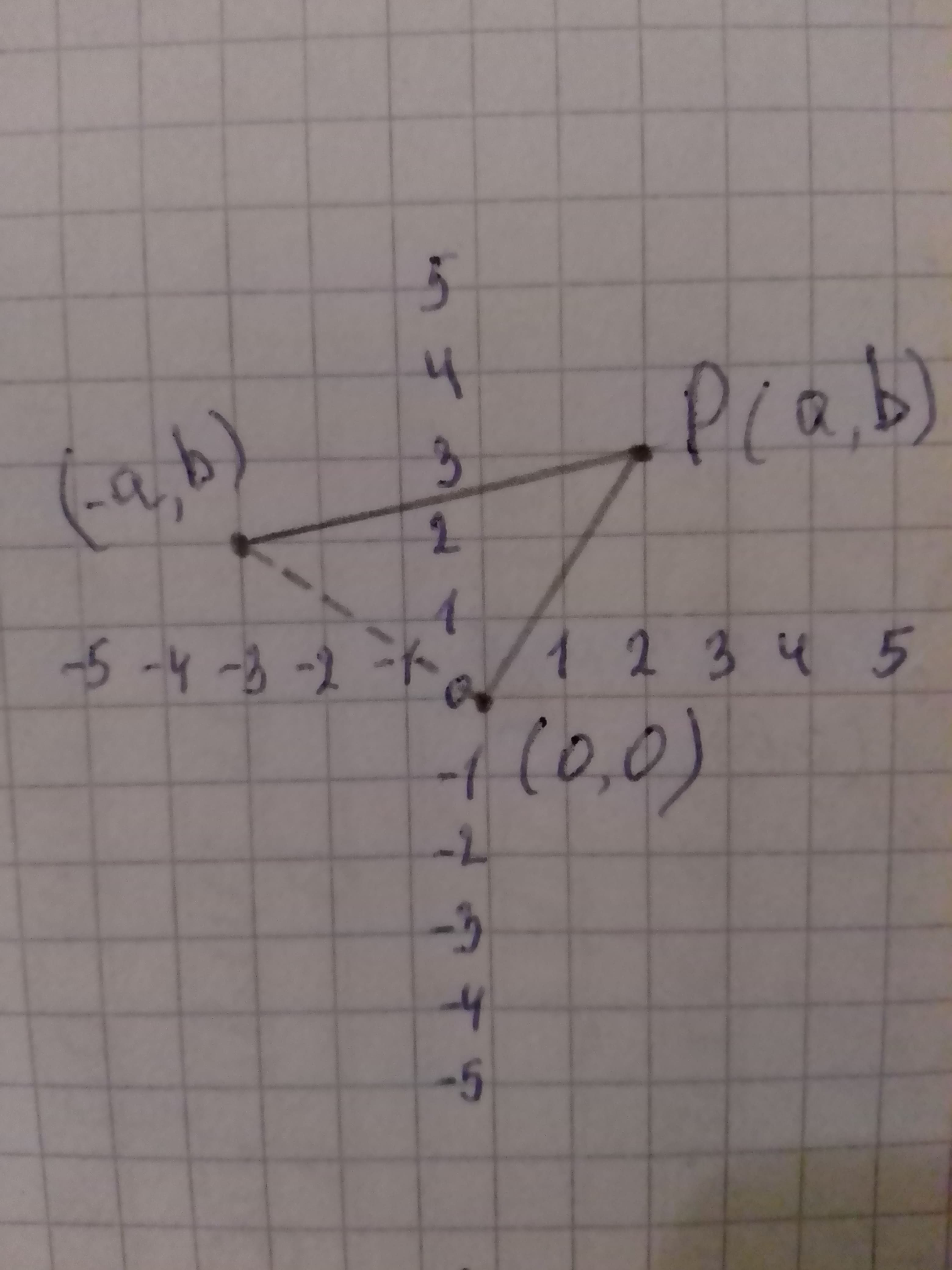Now, rotate the resulting figure to form the image of cockwise rotation as under: